# Locating an object - distance and displacementPage 1

#### WATCH ALL SLIDES

Slide 1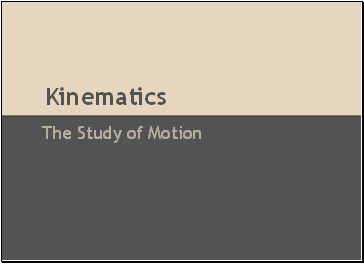Kinematics

The Study of Motion

Slide 2## Scalar and vector

I drove 125 kms and reached my destination

I drove 125 kms south and reached my destination

Slide 3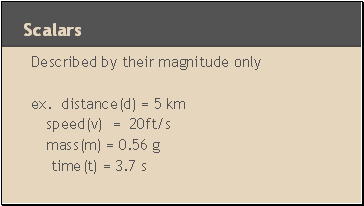## Scalars

Described by their magnitude only

ex. distance(d) = 5 km

speed(v) = 20ft/s

mass(m) = 0.56 g

time(t) = 3.7 s

Slide 4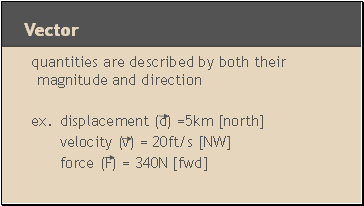## Vector

quantities are described by both their magnitude and direction

ex. displacement (d) =5km [north]

velocity (v) = 20ft/s [NW]

force (F) = 340N [fwd]

Slide 5## Distance and displacement

distance - how far an object has travelled from its original position

displacement - how far away an object is from its original position

Slide 6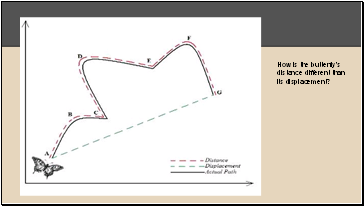How is the butterfly’s distance different than its displacement?

Slide 7What is the skiers distance and displacement at t=1min, t=2 min and t=3 min?

Slide 8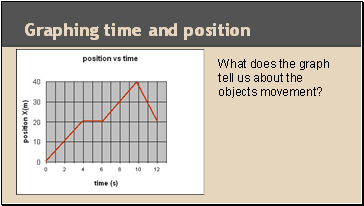## Graphing time and position

What does the graph tell us about the objects movement?

Slide 9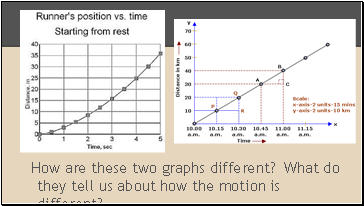How are these two graphs different? What do they tell us about how the motion is different?